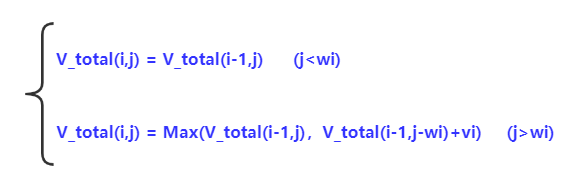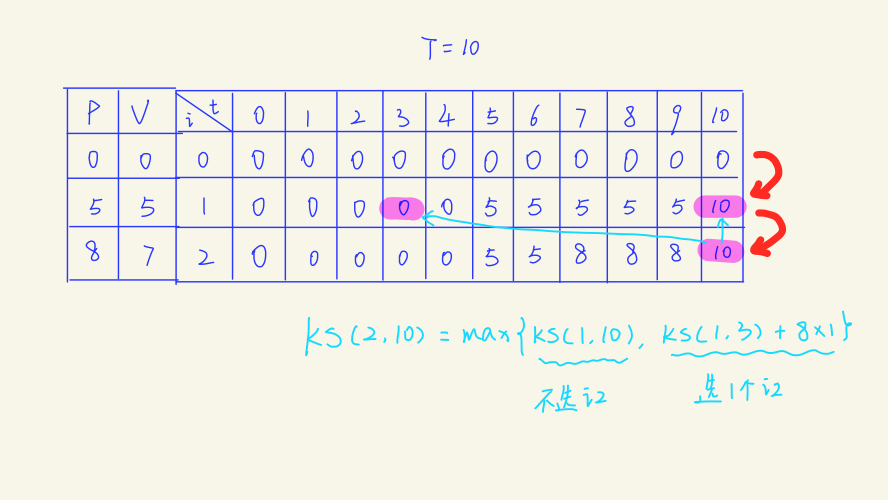Kubrnete109 1 0

## 问题描述## 完全背包的递推关系

V_total(i,j) = max{V_total(i-1, j - w[i] * k) + v[i] * k}; （0 <= k * w[i] <= j）

（注：当k=0则为不选，最多可选k=j/w[i]件。在这个范围中找出k种选择种的最优解）

int V_total(int i, int j)
{
int value = 0;
if (i && j) //可选物品个数和初始容量皆不为0
{
if (j < w[i])
value = V_total(i - 1, j);
else
for (int k = 0; k * w[i] <= j; k++)
{
int temp = V_total(i - 1, j - w[i] * k) + v[i] * k;
value = temp > value ? temp : value;
}
}
return value;
}

## 填表法i=1时，若t<10，背包最多只能放下1个物品1，价值为5；当t=10时，能放下2个物品1，因此i=1时的最大值为10。

i=2时，若5<=t<7时，背包能放下1个物品1；当7<=t<10时，背包能放下1个物品2，最大值为8；当t=10时，能放下2个物品1或1个物品2，因此最大值为10。通过求解这些子问题的最优解，我们推导出了全局最优解。

for (int i = 1; i <= n; i++)
for (int j = 1; j <= c; j++)
for (int k = 0; k * w[i] <= j; k++)
values[i + 1][j] = max(values[i + 1][j], values[i][j - k * w[i]] + k * v[i]);
cout << values[n][c];

V_total(t) = max{V_total(t), V_total(t - wi) + vi}

#include <bits/stdc++.h>
using namespace std;
int n, c, d;
int v, w, dp;
int main()
{

cin >> n >> c;
for (int i = 1; i <= n; i++)
cin >> w[i] >> v[i];
for (int i = 1; i <= n; i++)
for (int j = w[i]; j <= c; j++)
dp[j] = max(dp[j],dp[j - w[i]] + v[i]);
cout << dp[c];
}

## 总结

(建议仔细思考其中的区别)Python正则表达式 2021-03-13 17:43